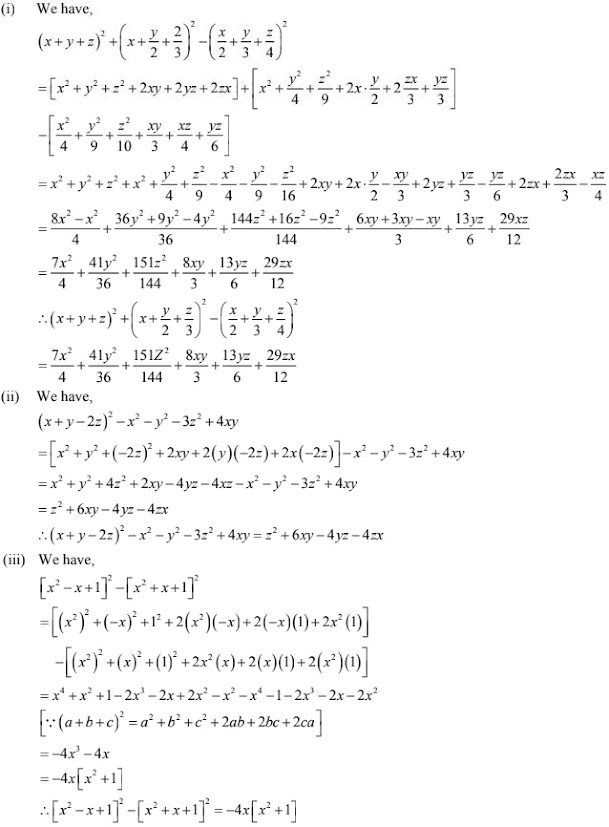# Chapter 4 Algebraic Identities RD Sharma Solutions Exercise 4.2 Class 9 Maths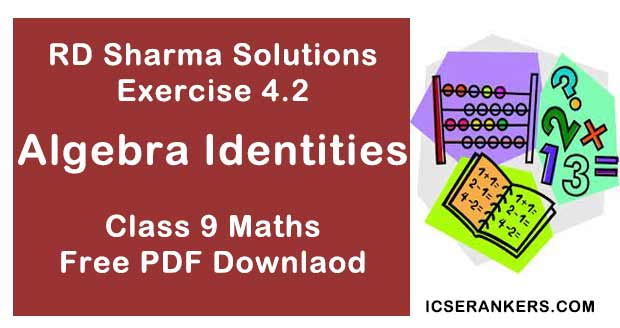Chapter Name RD Sharma Chapter 4 Algebraic Identities Exercise 4.2 Book Name RD Sharma Mathematics for Class 10 Other Exercises Exercise 4.1 Related Study NCERT Solutions for Class 10 Maths

### Exercise 4.2 Solutions

1. Write the following in the expanded form:
(i) (a + 2b + c)2
(ii) (2a - 3b - c)2
(iii) (-3x + y + z)2
(iv) (m + 2n - 5p)2
(v) (2 + x - 2y)2
(vi) (a2 + b2 + c2 )2
(vii) (ab + bc + ca)2
(viii) (x/y + y/z + z/x)2
(ix) (a/bc + b/ca + c/ab)2
(x) (x + 2y + 4z)2
(xi) (2x - y + z)2
(xii) (-2x + 3y + 2z)2

Solution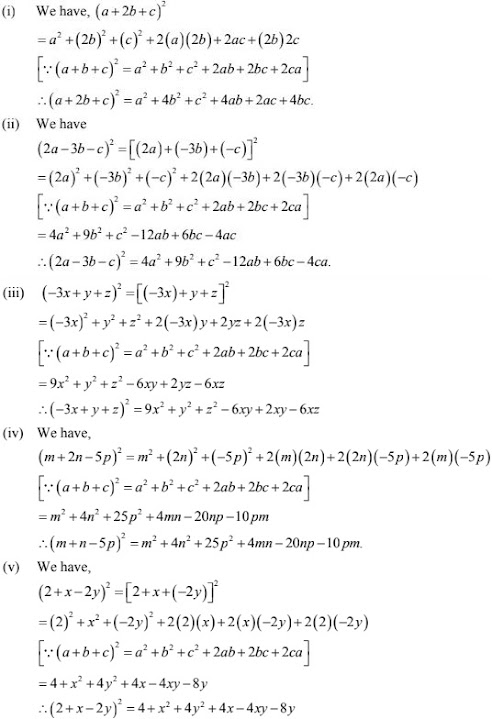2. Simplify :
(i) (a + b + c)2 + (a - b + c)2
(ii) (a + b + c)2 - (a - b + c)2
(iii) (a + b + c)2 + (a - b + c)2 + (a + b - c)2
(iv) (2x + p  - c)2 - (2x - p + c)2
(v) (x2 + y2 - z2 ) - (x2 - y2 + z2 )2
Solution
(i) We have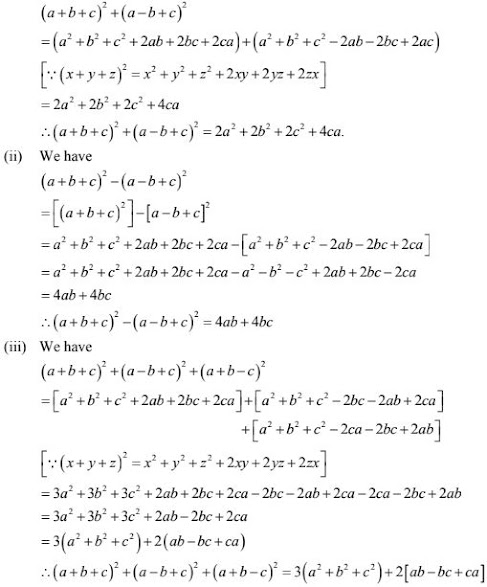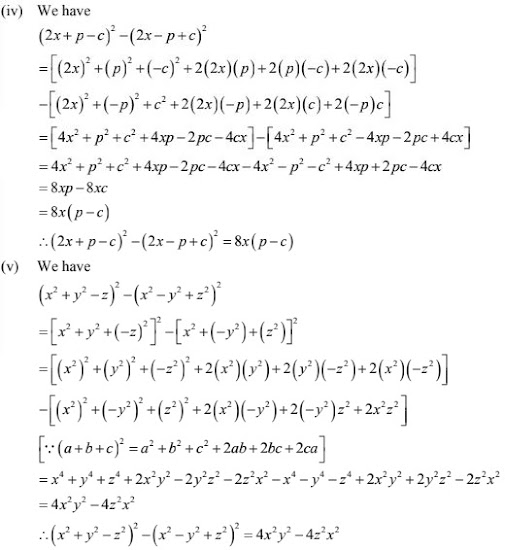3. If a + b + c = 0 and a2 + b2 + c2 = 16, find the value of ab + bc + ca.
Solution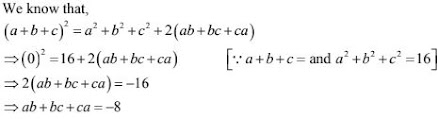4. If a2 + b2 + c2 = 16 and ab + bc + ca = 10, find the value of  a + b + c.
Solution
We know that,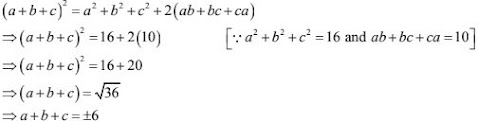5. If a + b + c = 9 and ab + bc + ca = 23, find the value of  a2 + b2 + c2
Solution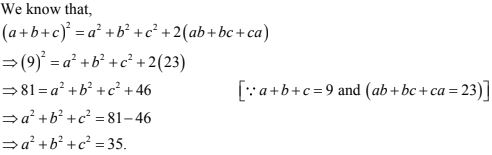6. Find the value of 4x2 + y2 + 25z2 + 4xy - 10yz - 20zx when x = 4, y = 3 and z = 2.
Solution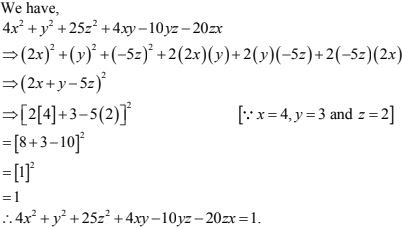7. Simplify each of the following expressions:
(i) (x + y +z)2 + (x + y/2 + 2/3)2 - (x/2 + y/3 + z/4)2
(ii) (x + y - 2z)2 - x2 - y2  -3z2  + 4xy
(iii) (x2 - x + 1)2 - (x2 + x + 1)2
Solution